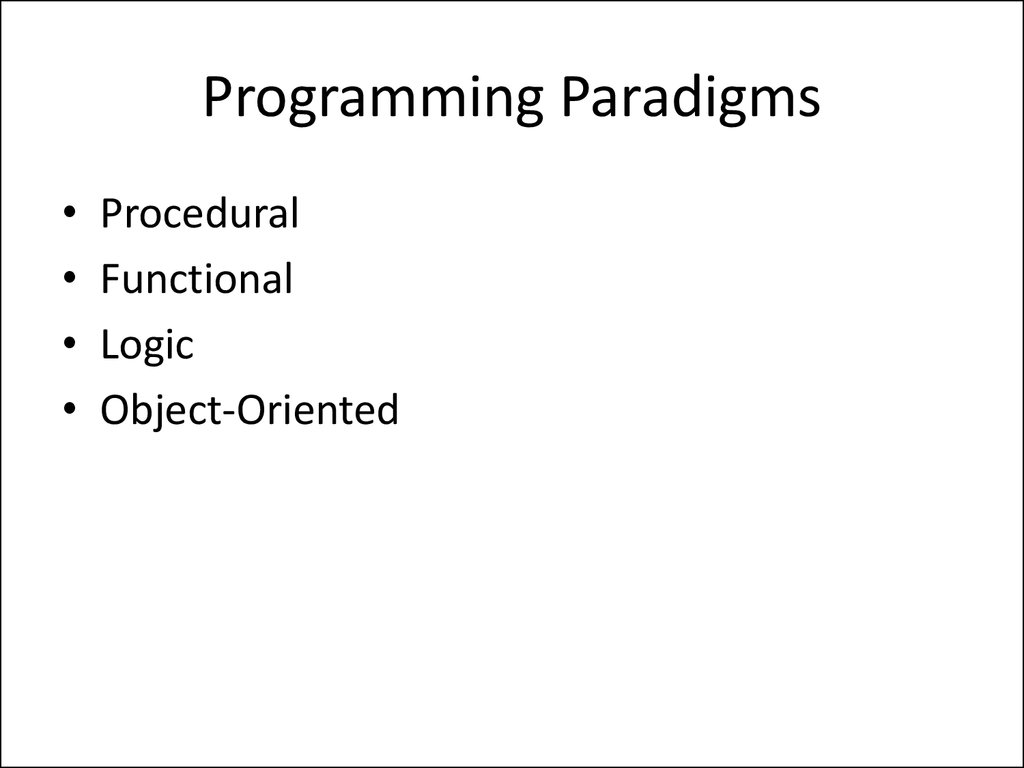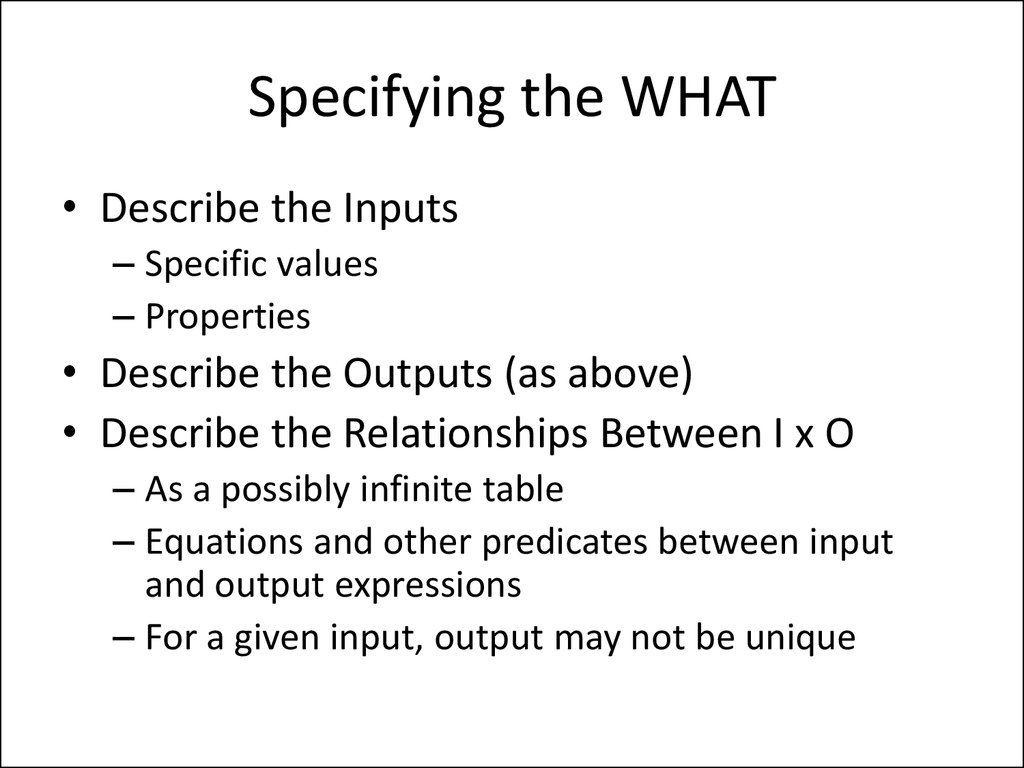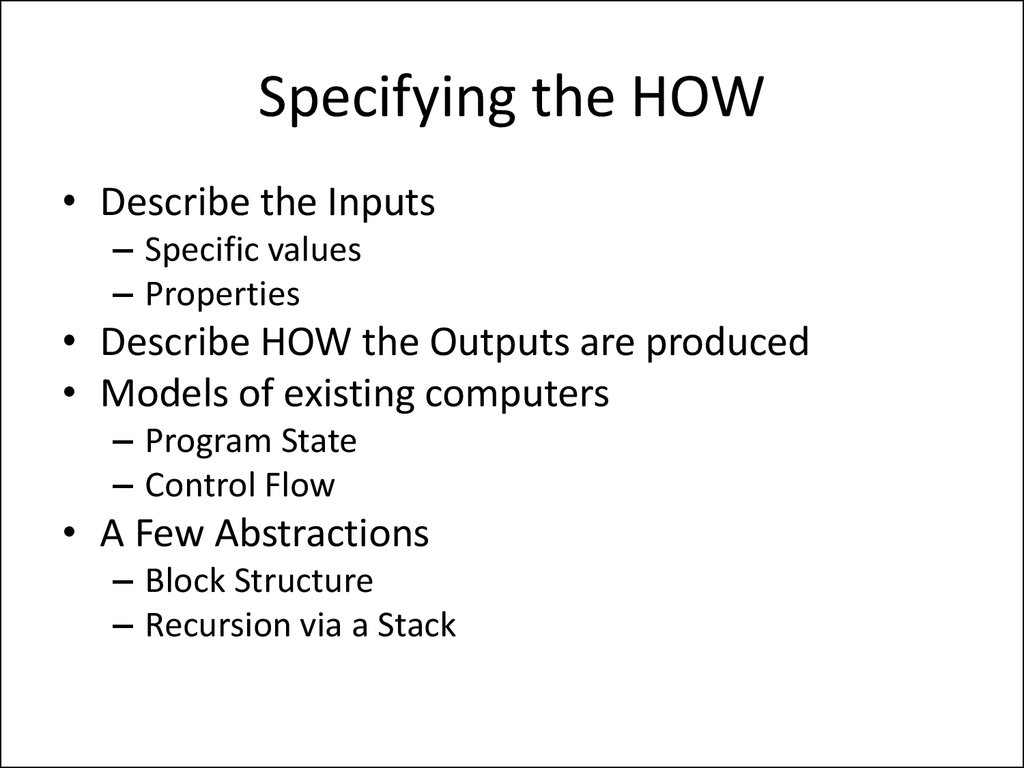Procedural
Functional
Logic
Object-Oriented

## 2. Specifying the WHAT

• Describe the Inputs
– Specific values
– Properties
• Describe the Outputs (as above)
• Describe the Relationships Between I x O
– As a possibly infinite table
– Equations and other predicates between input
and output expressions
– For a given input, output may not be unique

## 3. Specifying the HOW

• Describe the Inputs
– Specific values
– Properties
• Describe HOW the Outputs are produced
• Models of existing computers
– Program State
– Control Flow
• A Few Abstractions
– Block Structure
– Recursion via a Stack

## 4. Procedural programming

• Describes the details of HOW the results are to be obtained, in terms of
the underlying machine model.
• Describes computation in terms of
– Statements that change a program state
– Explicit control flow
• Synonyms
– Imperative programming
– Operational
• Fortran, C, …
– Abstractions of typical machines
– Control Flow Encapsulation
• Control Structures
• Procedures
– No return values
• Functions
– Return one or more values
• Recursion via stack

## 5. Procedural Programming: State

• Program State
– Collection of Variables and their values
– Contents of variables change
• Expressions
– Not expected to change Program State
• Assignment Statements
• Other Statements
• Side Effects

## 6. C, C++, C#, Java

• Abstractions of typical machines
• Control Flow Encapsulation
– Control Structures
– Procedures
• No return values
– Functions
• Return one or more values
– Recursion via stack
• Better Data Type support

## 7. Illustrative Example

• Expression (to be computed) : a + b + c
• Recipe for Computation
– Account for machine limitations
– Intermediate Location
• T := a + b;
T := T + c;
– Accumulator Machine
– Stack Machine

## 8. Declarative Programming

• Specifies WHAT is to be computed abstractly
• Expresses the logic of a computation without
describing its control flow
• Declarative languages include
– logic programming, and
– functional programming.
• often defined as any style of programming
that is not imperative.

## 9. Imperative vs Non-Imperative

• Functional/Logic style clearly separates WHAT
aspects of a program (programmers’
responsibility) from the HOW aspects
(implementation decisions).
• An Imperative program contains both the
specification and the implementation details,
inseparably inter-twined.

## 10. Procedural vs Functional

• Program: a sequence of
instructions for a von
Neumann m/c.
• Computation by
instruction execution.
• Iteration.
• Modifiable or updatable
variables..
• Program: a collection of
function definitions
(m/c independent).
• Computation by term
rewriting.
• Recursion.
• Assign-only-once
variables.

## 11. Functional Style : Illustration

• Definition: Equations
sumto(0) = 0
sumto(n) = n + sumto(n-1)
• Computation: Substitution and Replacement
sumto(2) = 2 + sumto (2-1)
= 2 + sumto(1)
= 2 + 1 + sumto(1-1) = 2 + 1 + sumto(0)
= 2+1+0=…
= 3

Imperative Style
tsum := 0;
i := 0;
while (i < n) do
i := i + 1;
tsum := tsum + I
od
Functional Style
func sumto(n: int): int;
if n = 0
then 0
else n + sumto(n-1)
fi
endfunc;
Storage efficient
No Side-effect

## 13. Bridging the Gap

• Imperative is not always faster, or more memory
efficient than functional.
• E.g., tail recursive programs can be automatically
translated into equivalent while-loops.
func xyz(n : int, r : int) : int;
if n = 0
then r
else xyz(n-1, n+r)
fi
endfunc

## 14. Analogy: Styles vs Formalisms

• Iteration
• Regular Expression
• Tail-Recursion
• Regular Grammar
• General Recursion
• Context-free Grammar

1.
2.
3.
4.
5.
6.
edge(a,b).
edge(a,c).
edge(c,a).
path(X,X).
path(X,Y) :- edge(X,Y).
path(X,Y) :- edge(X,Z), path(Z,Y).

## 16. Logic Programming

• A logic program defines a set of relations.
• This “knowledge” can be used in various ways
by the interpreter to solve different “queries”.
• In contrast, the programs in other languages
• Make explicit HOW the “declarative
knowledge” is used to solve the query.

## 17. Append in Prolog

append([], L, L).
append([ H | T ], X, [ H | Y ]) :append(T, X, Y).
Uses pattern matching.
– “[]” and “|” stand for empty list and cons
operation.

## 18. Different Kinds of Queries

• Verification
– append: list x list x list
• append(, [2,3], [1,2,3]).
• Concatenation
– append: list x list -> list
• append(, [2,3], R).

## 19. More Queries

• Constraint solving
– append: list x list -> list
• append( R, [2,3], [1,2,3]).
– append: list -> list x list
• append(A, B, [1,2,3]).
• Generation
– append: -> list x list x list
• append(X, Y, Z).

## 20. Object-Oriented Style

• Programming with Abstract Data Types
• Basic Program Unit: Class
• Abstraction enforced by encapsulation..
• Basic Run-time Unit: Object
– Instance of a class.
• Has an associated state.

## 21. Procedural vs Object-Oriented

• Emphasis on procedural
abstraction.
• Top-down design; Stepwise refinement.
• Suited for programming
in the small.
• Emphasis on data
abstraction.
• Bottom-up design;
Reusable libraries.
• Suited for programming
in the large.

## 22. Integrating Heterogeneous Data

• In C, Pascal, etc., use
• Union Type / Switch Statement
• Variant Record Type / Case Statement
• In C++, Java, Eiffel, etc., use
• Abstract Classes / Virtual Functions
• Interfaces and Classes / Dynamic Binding

## 23. Comparison : Figures example

• Data
– Square
• side
– Circle
• Operation (area)
– Square
• side * side
– Circle
• Classes
– Square
• side
• area
(= side * side)
– Circle
• area

## 24. Adding a new operation

• Data
...
• Operation (area)
• Operation (perimeter)
– Square
• 4 * side
– Circle
• 2 * PI * radius
• Classes
– Square
• ...
• perimeter
(= 4 * side)
– Circle
• ...
• perimeter
(= 2 * PI * radius)

## 25.

• Data
– ...
– rectangle
• length
• width
• Operation (area)
– ...
– rectangle
• length * width
• Classes
– ...
– rectangle
• length
• width
• area
(= length * width)

## 26. Procedural vs Object-Oriented

• New operations cause additive changes in
procedural style, but require modifications to
all existing “class modules” in object-oriented
style.
• New data representations cause additive
changes in object-oriented style, but require
modifications to all “procedure modules”.

## 27. Object-Oriented Concepts

• Data Abstraction (specifies behavior)
• Encapsulation (controls visibility of names)
• Polymorphism (accommodates various
implementations)
• Inheritance (facilitates code reuse)
• Modularity (relates to unit of compilation)

## 28. Example : Role of interface in decoupling

• Client
– Determine the number of elements in a collection.
• Suppliers
– Collections : Vector, String, List, Set, Array, etc
• Procedural Style
– A client is responsible for invoking appropriate supplier
function for determining the size.
• OOP Style
– Suppliers are responsible for conforming to the standard
interface required for exporting the size functionality to a
client.

## 29. Client in Scheme

• (define (size C)
(cond
( (vector? C) (vector-length C) )
( (pair? C) (length C) )
( (string? C) (string-length C) )
( else
“size not supported”) )))
• (size (vector 1 2 (+ 1 2)))
• (size ‘(one “two” 3))

## 30. Suppliers and Client in Java

Interface Collection {int size(); }
class myVector extends Vector
implements Collection {
}
class myString extends String
implements Collection {
public int size() { return length();}
}
class myArray implements Collection {
int[] array;
public int size() {return array.length;}
}
Collection c = new myVector(); c.size();